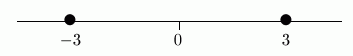﻿ Solving Simple Absolute Value Sentences
SOLVING SIMPLE ABSOLUTE VALUE SENTENCES
by Dr. Carol JVF Burns (website creator)
Follow along with the highlighted text while you listen!

Recall that $\,|x|\,$ gives the distance between $\,x\,$ and $\,0\,$.
If you think in terms of distance, then it's easy to solve sentences involving absolute value!

EXAMPLE: an absolute value equation
Solve: $|x| = 3$
$x = 3\ \text{ or }\ x=-3$
Note:
We want all numbers $\,x\,$ whose distance from zero is $\,3\,$.
$$\cssId{s16}{\overset{\text{whose distance from zero...}}{\overbrace{\ |\overset{\text{want numbers \,x\,...}}{\ \strut x\ }|\ }} \overset{\text{is...}}{\strut \ \ \ =\ \ \ } \overset{\text{three}}{\strut 3}}$$ The diagram above shows how the sentence is telling you what you want!
Interpret it in the following order:
 1) We want numbers $\,x\,$... (the unknown is $\,x\,$) 2) whose distance from zero... (the vertical bars, $|\ |$, ask for distance from zero) 3) is... (the equal sign) 4) $3$ three
Remember that you can ‘walk’ from zero in two directions: to the right, and to the left.
The number $\,3\,$ is three units from zero to the right;
the number $\,-3\,$ is three units from zero to the left.The word ‘or’ in the sentence ‘$\,x=3\text{ or }x=-3\,$’ is the mathematical word ‘or’.
You may want to review its meaning:
EXAMPLE: an absolute value inequality involving ‘less than’
Solve: $|x| \lt 3$
$-3 \lt x \lt 3$
Note:
We want all numbers $\,x\,$ whose distance from zero is less than $\,3\,$.
$$\cssId{s40}{\overset{\text{whose distance from zero...}}{\overbrace{\ |\overset{\text{want numbers \,x\,...}}{\ \strut x\ }|\ }} \overset{\text{is less than...}}{\strut \ \ \ \ \ \lt\ \ \ \ \ } \overset{\text{three}}{\ \ \ \ \strut 3\ \ \ \ }}$$ The diagram above shows how the sentence is telling you what you want!
Interpret it in the following order:
 1) We want numbers $\,x\,$... (the unknown is $\,x\,$) 2) whose distance from zero... (the vertical bars, $|\ |$, ask for distance from zero) 3) is less than... (the ‘less than’ symbol) 4) $3$ three
You can walk less than three units to the right—this gets you the numbers from $\,0\,$ to $\,3\,$.
You can walk less than three units to the left—this gets you the numbers from $\,0\,$ to $\,-3\,$.
Together, you end up with all the numbers between $\,-3\,$ and $\,3\,$:The sentence ‘$\,-3 \lt x \lt 3\,$’ is just a shorthand for ‘$\,-3\lt x\ \text{ and }\ x \lt 3\,$ ’.
That's the mathematical word ‘and’.
You may want to review its meaning:   Practice with the Mathematical Words ‘and’, ‘or’, ‘is equivalent to’

The shorthand ‘$\,-3\lt x\lt 3\,$’ is a great shorthand, because you see an $\,x\,$ trapped between $\,-3\,$ and $\,3\,$;
and those are precisely the values of $\,x\,$ that make the sentence true.
EXAMPLE: an absolute value inequality involving ‘greater than’
Solve: $|x| \gt 3$
$x \lt -3\ \text{ or }\ x\gt 3$
Note:
We want all numbers $\,x\,$ whose distance from zero is greater than $\,3\,$.
$$\cssId{s66}{\overset{\text{whose distance from zero...}}{\overbrace{\ |\overset{\text{want numbers \,x\,...}}{\ \strut x\ }|\ }} \overset{\text{is greater than...}}{\strut \ \ \ \ \ \gt\ \ \ \ \ } \overset{\text{three}}{\ \ \ \ \strut 3\ \ \ \ }}$$ The diagram above shows how the sentence is telling you what you want!
Interpret it in the following order:
 1) We want numbers $\,x\,$... (the unknown is $\,x\,$) 2) whose distance from zero... (the vertical bars, $|\ |$, ask for distance from zero) 3) is greater than... (the ‘greater than’ symbol) 4) $3$ three
You can walk more than three units to the right—this gets you all the numbers to the right of $\,3\,$.
You can walk more than three units to the left—this gets you all the numbers to the left of $\,-3\,$.
Together, you end up with the two pieces shown below:The word ‘or’ in the sentence ‘$\,x\lt -3\ \text{ or }\ x\gt 3\,$’ is the mathematical word ‘or’.
You may want to review its meaning:
EXAMPLE: an absolute value sentence with no solutions
Solve: $|x| \lt -1$
Solution:
There are no solutions, since distance can't be negative.
That is, $\,|x|\,$ is always greater than or equal to zero; so, it can't ever be less than $\,-1\,$.
EXAMPLE: an absolute value sentence that is always true
Solve: $|x| \gt -1$
Solution:
All real numbers are solutions, since all distances are nonnegative.
That is, $\,|x|\,$ is always greater than or equal to zero; so, it must also be greater than $\,-1\,$.
Master the ideas from this section

When you're done practicing, move on to:
Solving Sentences Involving ‘Plus or Minus’

Solve the given absolute value sentence.
Write the result in the most conventional way.

For more advanced students, a graph is displayed.
For example, the equation $\,|x| = 3\,$
is optionally accompanied by the graph of $\,y = |x|\,$ (the left side of the equation, dashed green)
and the graph of $\,y = 3\,$ (the right side of the equation, solid purple).
In this example, you are finding the values of $\,x\,$ where the green graph intersects the purple graph.
Click the “show/hide graph” button if you prefer not to see the graph.

CONCEPT QUESTIONS EXERCISE: